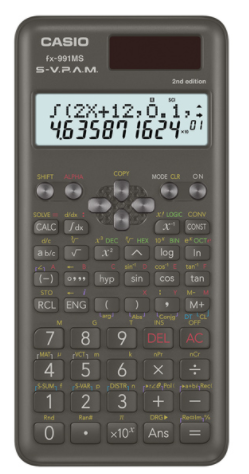# Casio FX-991MS-2 2nd Edition Non Programmable Scientific Calculator #C77B*Image may differ with actual product's layout, color, size & dimension. No claim will be accepted for image mismatch.

# Casio FX-991MS-2 2nd Edition Non Programmable Scientific Calculator #C77B

Product Id: 130.01.685.48

Regular Price  1,360
Special Price  1,300

Delivery time?

Quick Overview

Model - Casio FX-991MS-2
Brand - Casio
Type - Non Programmable Scientific Calculator
Display - Dot matrix display
Digit - 12 digits (10 + 2 digits)
Key - Plastic keys
Model
Casio FX-991MS-2
Type
Non Programmable Scientific Calculator
Display
Dot matrix display
Digit
12 digits (10 + 2 digits)
Key
Plastic keys
Number of Functions
401
Power Sources
Solar & Battery
Dimensions
161.5 x 77 x 11.1mm
Weight (Kg)
95gm
Specialty
2nd Edition Non Programmable Scientific Calculator, Solar & Battery, Solar powered when light is sufficient, battery powered when light is insufficient, Fraction calculations, Combination and permutation, Statistics (STAT-data editor, Standard deviation, Regression analysis), 9 variable memories, Comes with slide-on hard case, 10 + 2 digits, Dot matrix display, Regular percent, Multi-replay, Plastic keys, S-V.P.A.M. (Super Visually Perfect Algebraic Method), All this helps to make mathematics easier to use and easier to understand than ever before, STAT-data editor, Vector, Scientific Constants, Base-n calculation, Basic Mathematical Functions, Basic Statistics, Metric conversion function, Non Graphing, Matrix, Equation, Integration, Complex number calculation, Differentiation
Others
Part No: C77B, 10 + 2 digits: 10-digit mantissa + 2-digit exponential display, Dot matrix display: High-resolution screen provides beautiful looking graphs every time, Regular percent: Regular percentage calculations, Multi-replay: Quick and easy recall of previously executed formulas for editing and re-execution, Solar & Battery: Solar powered when light is sufficient, battery powered when light is insufficient, Plastic keys: Designed and engineered for easy operation, S-V.P.A.M. (Super Visually Perfect Algebraic Method): All the features of the existing V.P.A.M. series plus a new 2-line display and a useful Replay function, STAT-data editor: Back-step viewing and editing of input data, Scientific Constants: can use constant symbols used in physics, science classes, Base-n calculation: Binary/Octal/Hexadecimal, Basic Mathematical Functions: Trigonometric, Exponential logarithmic, etc., Basic Statistics: Standard statistics functions such as Mean, SUM, Standard Deviation, and Regression, Metric conversion function: can convert a number in one unit to a number in a different unit
Warranty
3 year
Made in/ Assemble
China
Country Of Origin
Japan

# fx-991MS2nd edition## Features

• Fraction calculations
• Combination and permutation
• Statistics (STAT-data editor, Standard deviation, Regression analysis)
• 9 variable memories
• Comes with slide-on hard case

### fx-82MS/85MS/350MS functions, in addition to:

• Equation calculations
• Integration/differential calculations
• Base-n calculations/conversions
• Complex number calculations
• Matrix calculations
• Vector calculations
• 40 scientific constants

Model - Casio FX-991MS-2, Brand - Casio, Type - Non Programmable Scientific Calculator, Display - Dot matrix display, Digit - 12 digits (10 + 2 digits), Number of Functions - 401, Key - Plastic keys, Power source - Solar & Battery, Dimension - 161.5 x 77 x 11.1mm, Weight - 95gm, Others - Part No: C77B, 10 + 2 digits: 10-digit mantissa + 2-digit exponential display, Dot matrix display: High-resolution screen provides beautiful looking graphs every time, Regular percent: Regular percentage calculations, Multi-replay: Quick and easy recall of previously executed formulas for editing and re-execution, Solar & Battery: Solar powered when light is sufficient, battery powered when light is insufficient, Plastic keys: Designed and engineered for easy operation, S-V.P.A.M. (Super Visually Perfect Algebraic Method): All the features of the existing V.P.A.M. series plus a new 2-line display and a useful Replay function, STAT-data editor: Back-step viewing and editing of input data, Scientific Constants: can use constant symbols used in physics, science classes, Base-n calculation: Binary/Octal/Hexadecimal, Basic Mathematical Functions: Trigonometric, Exponential logarithmic, etc., Basic Statistics: Standard statistics functions such as Mean, SUM, Standard Deviation, and Regression, Metric conversion function: can convert a number in one unit to a number in a different unit, Specialty - 2nd Edition Non Programmable Scientific Calculator, Solar & Battery, Solar powered when light is sufficient, battery powered when light is insufficient, Fraction calculations, Combination and permutation, Statistics (STAT-data editor, Standard deviation, Regression analysis), 9 variable memories, Comes with slide-on hard case, 10 + 2 digits, Dot matrix display, Regular percent, Multi-replay, Plastic keys, S-V.P.A.M. (Super Visually Perfect Algebraic Method), All this helps to make mathematics easier to use and easier to understand than ever before, STAT-data editor, Vector, Scientific Constants, Base-n calculation, Basic Mathematical Functions, Basic Statistics, Metric conversion function, Non Graphing, Matrix, Equation, Integration, Complex number calculation, Differentiation, Warranty - 3 year, Country of Origin - Japan, Made in/ Assemble - China

## Your review## Your Question